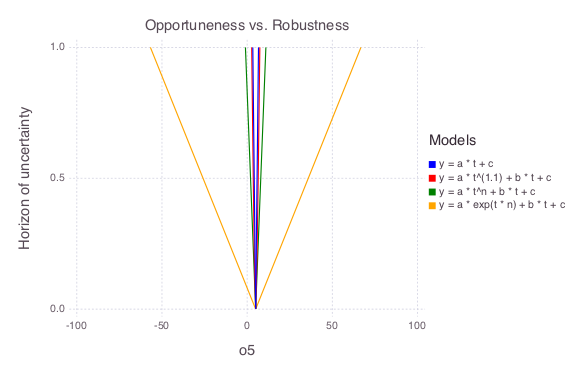Skip to content

# Information Gap Decision Analysis

## Information Gap Analysis

All the figures below are generated using examples/model_analysis/infogap.jl.

### Setup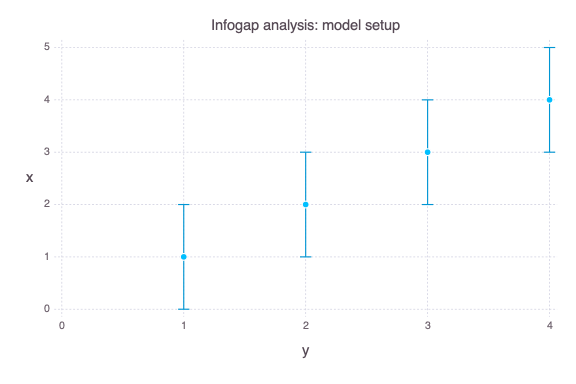• There are 4 uncertain observations at times t = [1,2,3,4]

• There are 4 possible models that can be applied to match the data

1. y(t) = a * t + c

2. y(t) = a * t^(1.1) + b * t + c

3. y(t) = a * t^n + b * t + c

4. y(t) = a * exp(t * n) + b * t + c

• There are 4 unknown model parameters with uniform prior probability functions:

1. a = Uniform(-10, 10)

2. b = Uniform(-10, 10)

3. c = Uniform(-5, 5)

4. n = Uniform(-3, 3)

• The model prediction for t = 5 is unknown and information gap prediction uncertainty needs to be evaluated

• The horizon of information gap uncertainty h is applied to define the acceptable deviations in the 4 uncertain observations.

### Infogap in model y(t) = a * t + c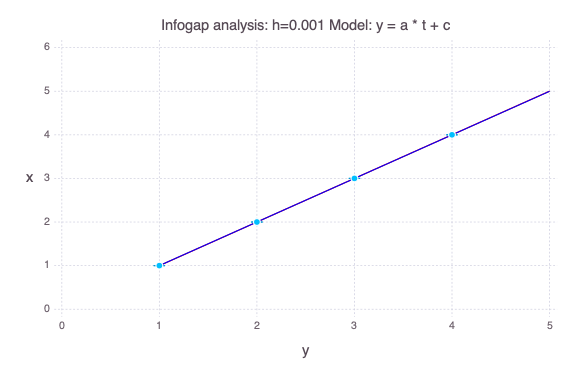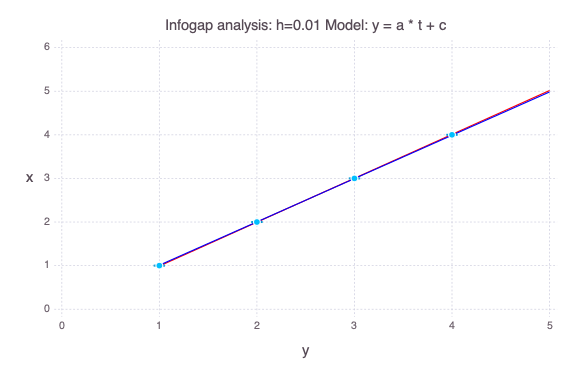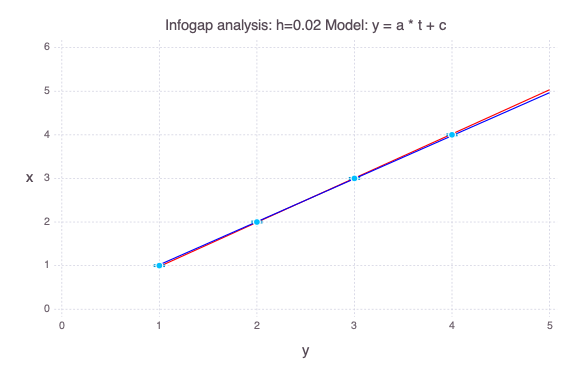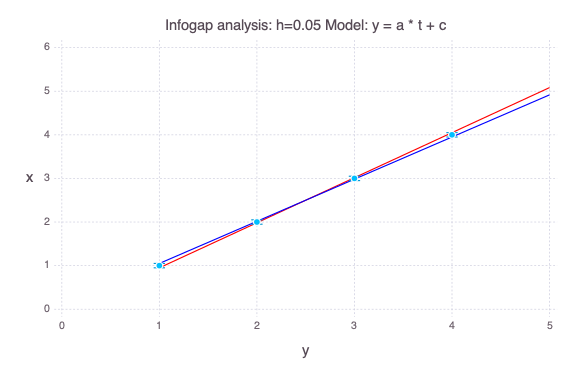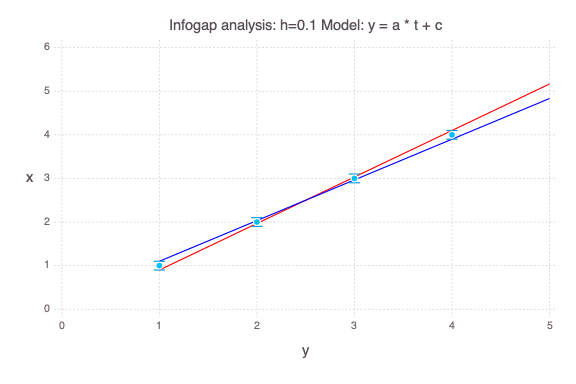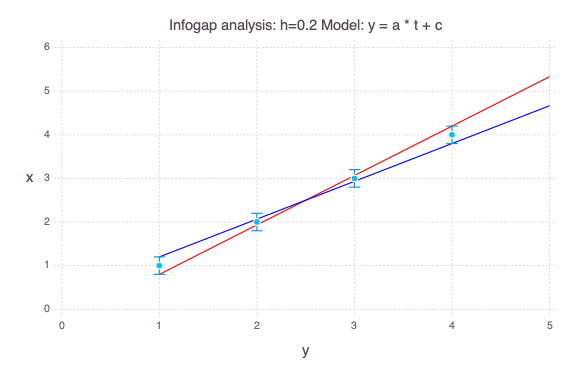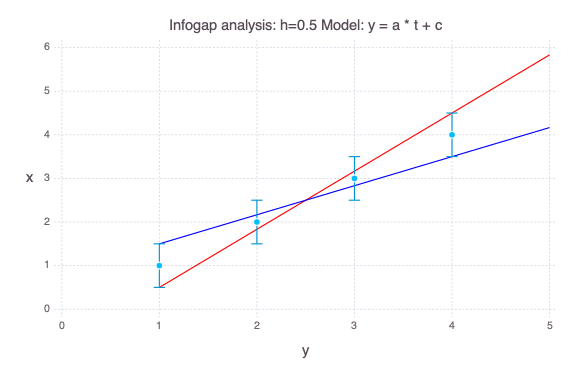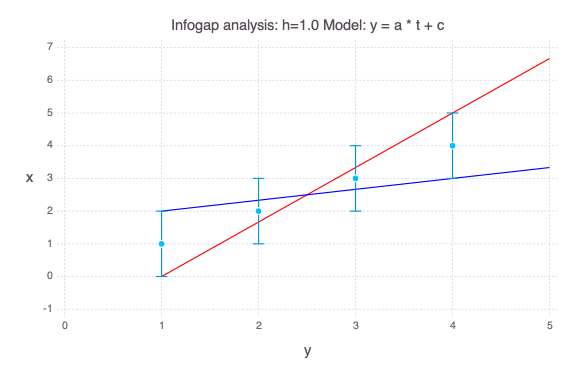### Infogap in model y(t) = a * t^(1.1) + b * t + c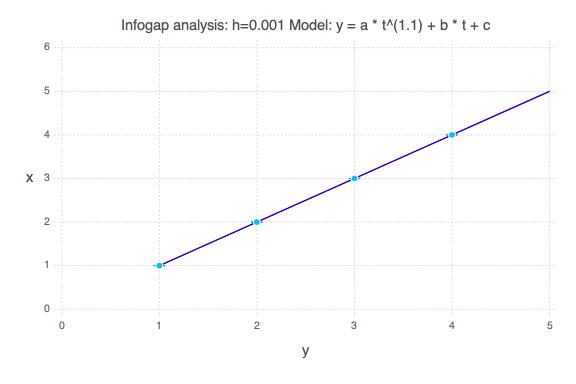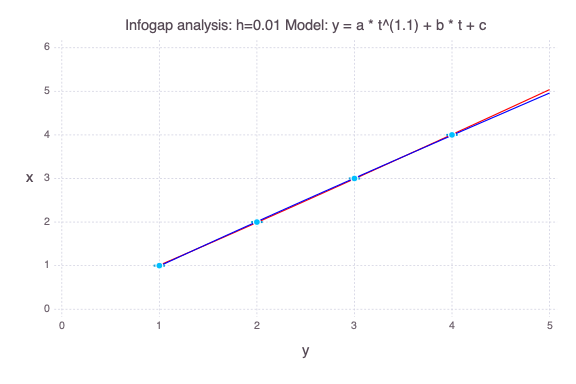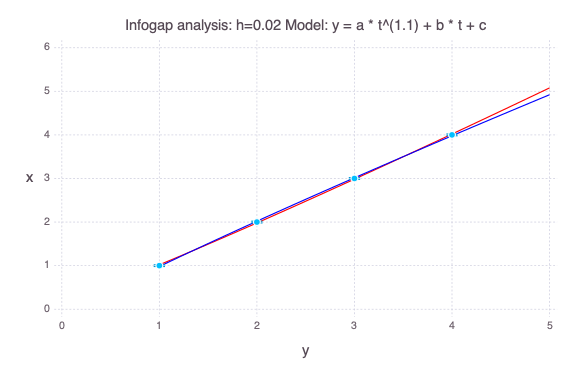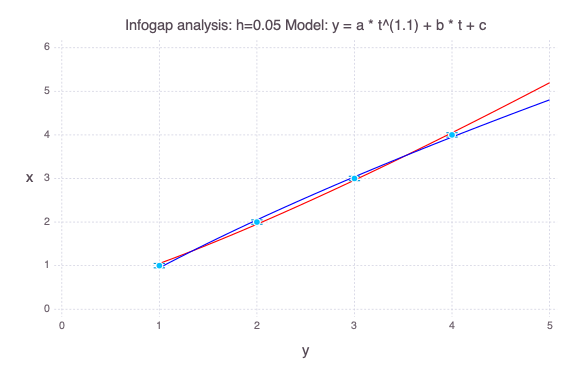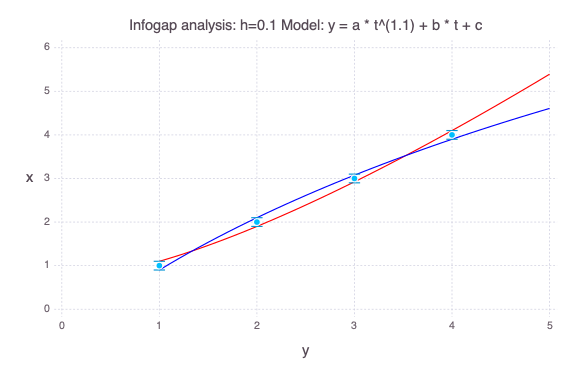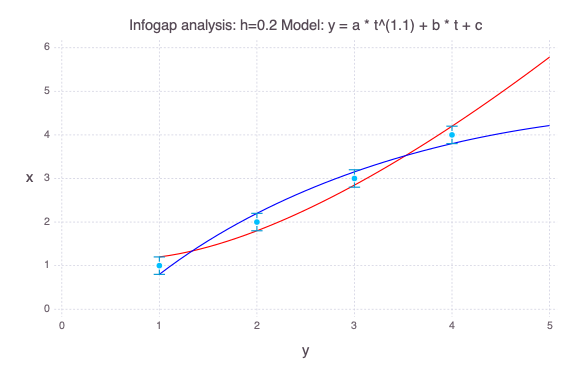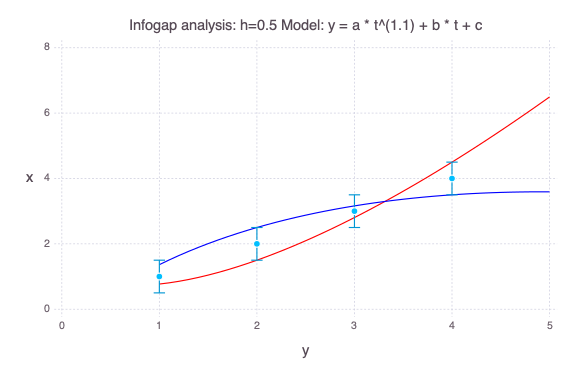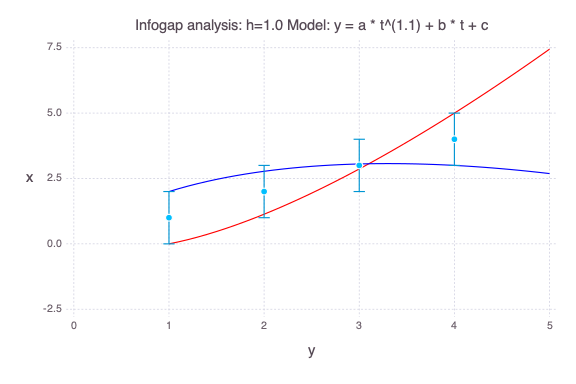### Infogap in model y(t) = a * t^n + b * t + c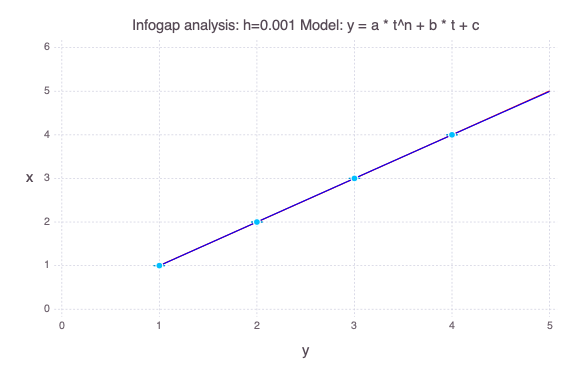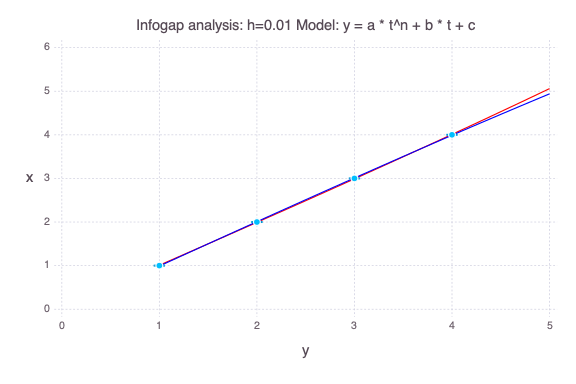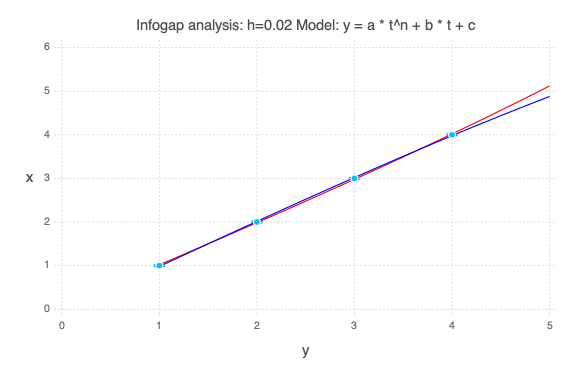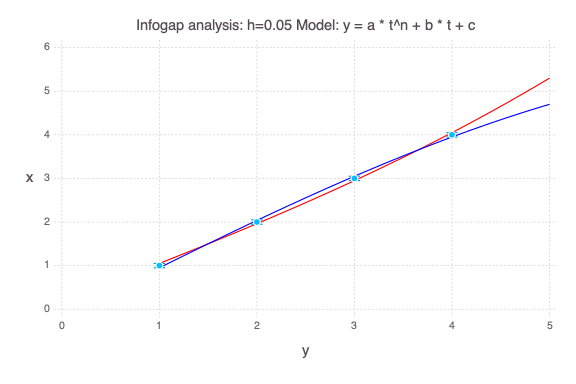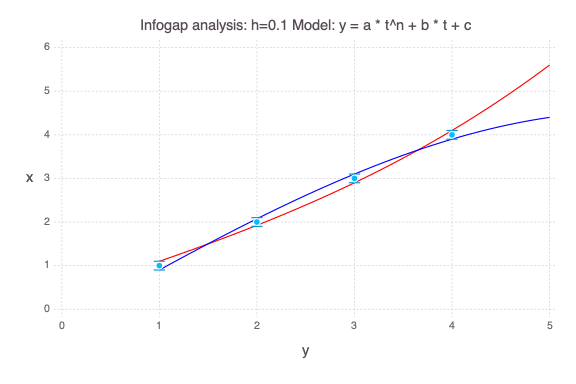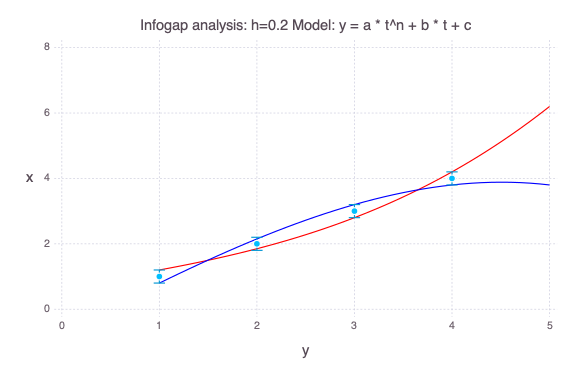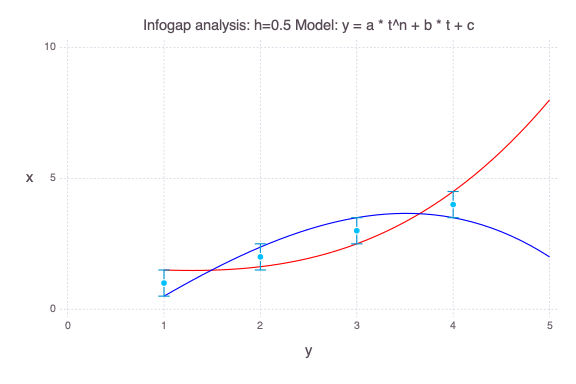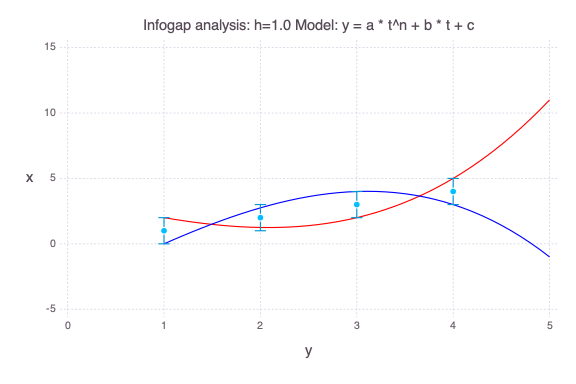### Infogap in model y(t) = a * exp(t * n) + b * t + c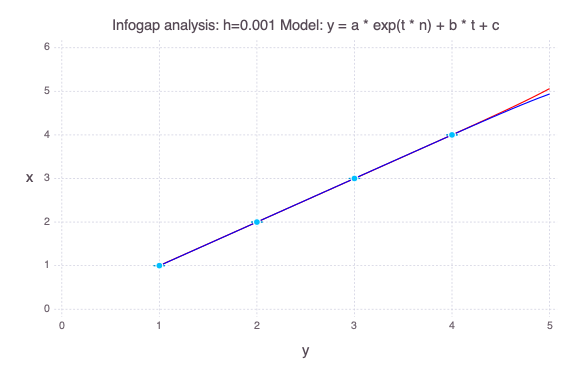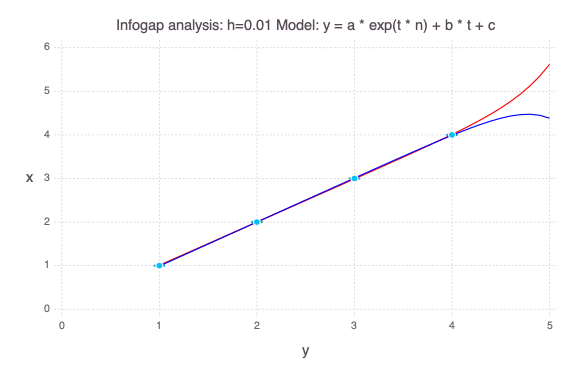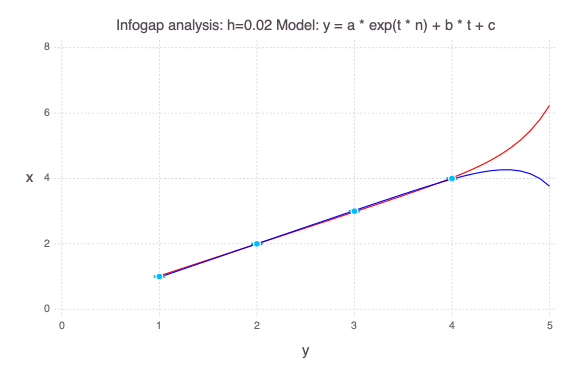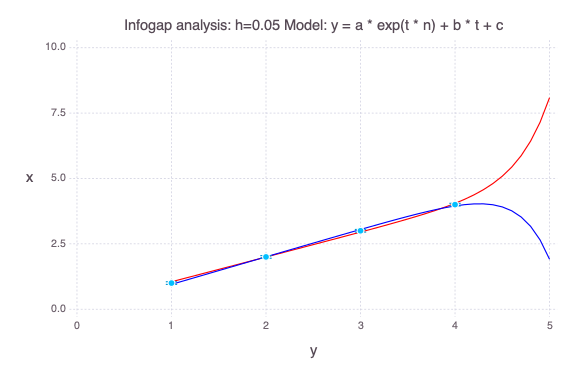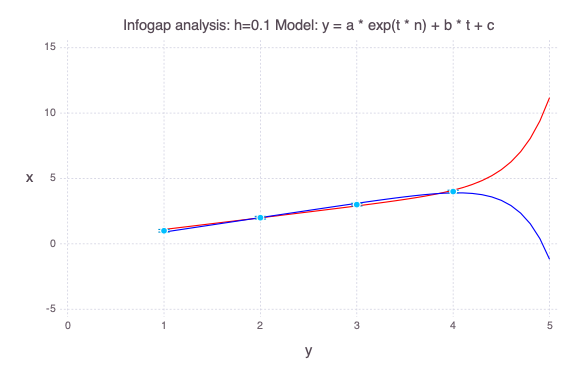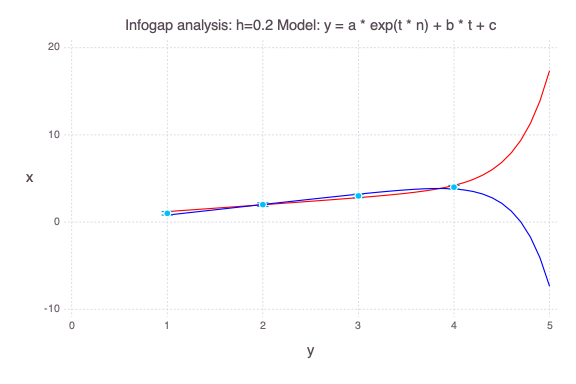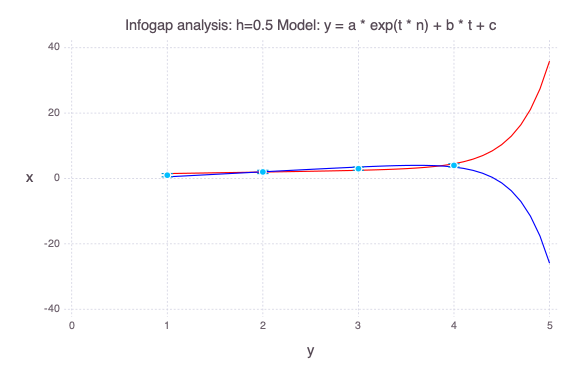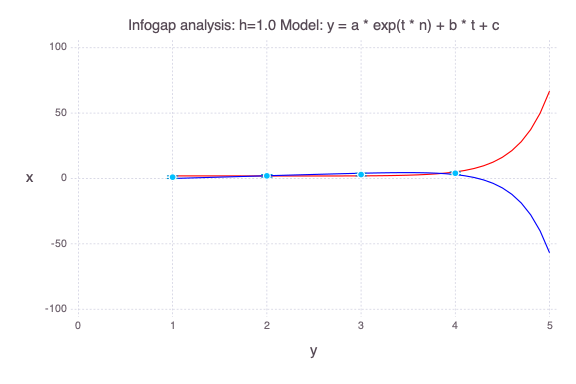### Opportuneness and Robustness of the 4 models